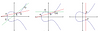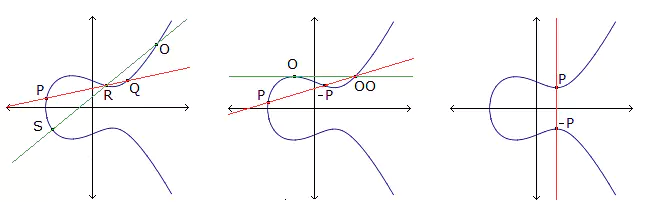# New upper bounds on the smallest size of a saturating set in a projective plane

### Journal article

D. Bartoli, A. Davydov, M. Giulietti, S. Marcugini, F. Pambianco
2016 XV International Symposium Problems of Redundancy in Information and Control Systems (REDUNDANCY), 2015

Semantic Scholar ArXiv DOI
Cite

### Cite

APA
Bartoli, D., Davydov, A., Giulietti, M., Marcugini, S., & Pambianco, F. (2015). New upper bounds on the smallest size of a saturating set in a projective plane. 2016 XV International Symposium Problems of Redundancy in Information and Control Systems (REDUNDANCY).

Chicago/Turabian
Bartoli, D., A. Davydov, M. Giulietti, S. Marcugini, and F. Pambianco. “New Upper Bounds on the Smallest Size of a Saturating Set in a Projective Plane.” 2016 XV International Symposium Problems of Redundancy in Information and Control Systems (REDUNDANCY) (2015).

MLA
Bartoli, D., et al. “New Upper Bounds on the Smallest Size of a Saturating Set in a Projective Plane.” 2016 XV International Symposium Problems of Redundancy in Information and Control Systems (REDUNDANCY), 2015.

## Abstract

In a projective plane Π<sub>q</sub> (not necessarily Desar-guesian) of order q, a point subset S is saturating (or dense) if any point of Π<sub>q</sub>\S is collinear with two points in S. Using probabilistic methods, more general than those previously used for saturating sets, the following upper bound on the smallest size s(2, q) of a saturating set in Π<sub>q</sub> is proved: s(2, q) <; 2√(q + 1)ln(q + 1) + 2 ~ 2√q ln q. We also show that for any constant c > 1 a random point set of size k in Π<sub>q</sub> with 2c√(q + 1) ln(q + 1) + 2 <; k <; q<sup>2</sup>-1/q+2 ~ q is a saturating set with probability greater than 1 - 1/(q + 1)<sup>2c2 -2</sup>. Our probabilistic approach is also applied to multiple saturating sets. A point set S ⊂ Π<sub>q</sub> is (1,μ)-saturating if for every point Q of Π<sub>q</sub>\S the number of secants of S through Q is at least μ, counted with multiplicity. The multiplicity of a secant l is computed as (<sup>#(l∩S)</sup><sub>2</sub>). The following upper bound on the smallest size s<sub>μ</sub>(2, q) of a (1,μ)-saturating set in Π<sub>q</sub> is proved: s<sub>μ</sub>(2, q) <; 2(μ + 1)√(q + 1)ln(q + 1) + 2 for 2 <; μ <; √q. By using inductive constructions, upper bounds on the smallest size of a saturating set (as well as on a (1, μ)-saturating set) in the projective space PG(N, q) are obtained. All the results are also stated in terms of linear covering codes.

Share